Get inspired by the success stories of our students in IIT JAM MS, ISI  MStat, CMI MSc DS.  Learn More

# Elchanan Mossel's Dice Paradox | ISI MStat 2018 PSB Problem 6This problem from ISI MStat 2018 PSB (Problem 6) is called the Elchanan Mossel's Dice Paradox. The problem has a paradoxical nature, but there is always a way out.

## Problem

A fair 6 -sided die is rolled repeatedly until a 6 is obtained. Find the expected number of rolls conditioned on the event that none of the rolls yielded an odd number.

### Prerequisites

• Geometric Distribution
• Conditional Probabilty
• Conditional Expectation
• Expectation Smooting Property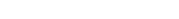• Common Sense

## Solution

### The Wrong Solution

Let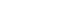be the throws of a die. Let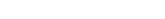Then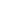~ Geo(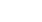)

But, here it is given that none of the rolls are odd numbers. So,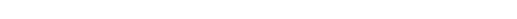Then~ Geo() Since, there are three posibilities in the reduced (conditional) sample space.

So,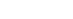.

Obviously, this is false. But you are not getting why it is false right? Scroll Down!

## Where it went wrong?

It went wrong in observing the given condition of the problem. Observe that it is given that none of the rolls are odd till the roll you got success, not for all the rolls beyond that also.

So,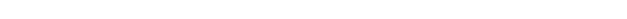So, we are essentially seeing that the sample space didn't get reduced all along, it got reduced till that point of the roll. This is where the paradox marches in.

We are thinking of the experiment as we are picking up only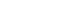in the experiment and rolling. No!

## The Elegant One Liner Solution

The idea is to think from a different perspective as with the case of every elegant solution. Let's reconstruct the experiment in a different way. It is like the following. Remember, we need to exclude the odd numbers, so just throw them away and start anew.

Idea

If you get, start counting the number of rolls again from the beginning. Stop when you get 6. This is the exact formulation of the waiting time to get a 6 without getting any odd numbers till that toss. We will show that our success is when we get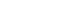in this experiment.

Mathematical Form

Let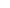be the time required to get an outcome different from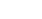Then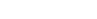is independent of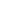for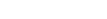because it is same for all. Thus, by the smooting property of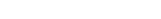.

Observe,~ Geo(). Hence,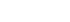.

## The Bigger Bash SolutionWe need to calculate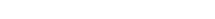.

For that we need to find out the conditional probabilities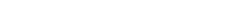and that is given by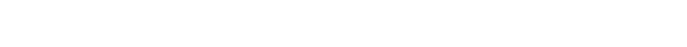where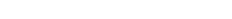. Thus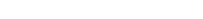follows a geometric distribution with parameterand consequently its expectation is.

Stay Tuned! Stay Blessed!

### Simulation in Python

import random

times = 0 #number of times a successful (all-even) sequence was rolled
rolls = 0 #total of all number of rolls it took to get a 6, on successful sequences
curr = 0
alleven = True

for x in range(0, 100000):

num = random.randint(1,6)
if num % 2 != 0:
alleven = False
else:
if num == 6:
if alleven:
times += 1
rolls += curr + 1
curr = 0
alleven = True
else:
curr += 1

print(rolls * 1.0 / times)
#1.51506456241

Source: mathstackexachange

Stay Tuned! Stay Blessed!

This problem from ISI MStat 2018 PSB (Problem 6) is called the Elchanan Mossel's Dice Paradox. The problem has a paradoxical nature, but there is always a way out.

## Problem

A fair 6 -sided die is rolled repeatedly until a 6 is obtained. Find the expected number of rolls conditioned on the event that none of the rolls yielded an odd number.

### Prerequisites

• Geometric Distribution
• Conditional Probabilty
• Conditional Expectation
• Expectation Smooting Property• Common Sense

## Solution

### The Wrong Solution

Letbe the throws of a die. LetThen~ Geo()

But, here it is given that none of the rolls are odd numbers. So,Then~ Geo() Since, there are three posibilities in the reduced (conditional) sample space.

So,.

Obviously, this is false. But you are not getting why it is false right? Scroll Down!

## Where it went wrong?

It went wrong in observing the given condition of the problem. Observe that it is given that none of the rolls are odd till the roll you got success, not for all the rolls beyond that also.

So,So, we are essentially seeing that the sample space didn't get reduced all along, it got reduced till that point of the roll. This is where the paradox marches in.

We are thinking of the experiment as we are picking up onlyin the experiment and rolling. No!

## The Elegant One Liner Solution

The idea is to think from a different perspective as with the case of every elegant solution. Let's reconstruct the experiment in a different way. It is like the following. Remember, we need to exclude the odd numbers, so just throw them away and start anew.

Idea

If you get, start counting the number of rolls again from the beginning. Stop when you get 6. This is the exact formulation of the waiting time to get a 6 without getting any odd numbers till that toss. We will show that our success is when we getin this experiment.

Mathematical Form

Letbe the time required to get an outcome different fromThenis independent offorbecause it is same for all. Thus, by the smooting property of.

Observe,~ Geo(). Hence,.

## The Bigger Bash SolutionWe need to calculate.

For that we need to find out the conditional probabilitiesand that is given bywhere. Thusfollows a geometric distribution with parameterand consequently its expectation is.

Stay Tuned! Stay Blessed!

### Simulation in Python

import random

times = 0 #number of times a successful (all-even) sequence was rolled
rolls = 0 #total of all number of rolls it took to get a 6, on successful sequences
curr = 0
alleven = True

for x in range(0, 100000):

num = random.randint(1,6)
if num % 2 != 0:
alleven = False
else:
if num == 6:
if alleven:
times += 1
rolls += curr + 1
curr = 0
alleven = True
else:
curr += 1

print(rolls * 1.0 / times)
#1.51506456241

Source: mathstackexachange

Stay Tuned! Stay Blessed!

This site uses Akismet to reduce spam. Learn how your comment data is processed.

### Knowledge Partner